# Problem 53745. Easy Sequences 60: Almost Cube Root

The almost cube root of an integer x, is the largest possible integer r, such that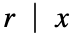and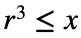. For example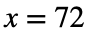, then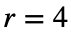, since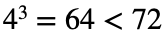and since the next larger divisor of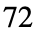which is 6, does not qualify because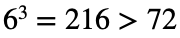. Obviously, if x is a perfect cube, then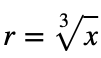.
Given an integer n, please find sum of the "almost cube roots" of all integers from 1 to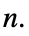For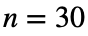, the program ouput should be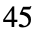:where: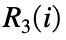is the almost cube root of i.

### Solution Stats

71.43% Correct | 28.57% Incorrect
Last Solution submitted on Aug 05, 2023

### Community Treasure Hunt

Find the treasures in MATLAB Central and discover how the community can help you!

Start Hunting!